# The Macropattern of Urbanization over the Course of the Last 5000 Years of World-System History

Author: Harper, Tony
Journal: Social Evolution & History. Volume 9, Number 1 / March 2010

Abstract

A mathematical model, Cmaxγ – Cmax – (γ – 1)T = 0, is constructed which reveals the macropattern of urbanization over the course of world-system history, i.e. the last 5000 years. When both unscaled and scaled sets of data are considered the detail of world-system urbanization reveals episodic or periodic behavior and the impact of urbanization over the last two hundred years becomes apparent. This periodic behavior is apparent when either urbanization is considered alone or in concert with changes in total world-system population. Further, the periodic behavior can be separated into both periods of urbanization and deurbanization and also periods of oscillatory or search-pattern behavior and periods of continuous change. The impact of change in γ has the greatest effect on the system.

Introduction

The phenomenon of urbanization first appears in the archeological record some 8–10,000 YBP. Since its inception, urbanization has accumulated an increasing portion of the world-system population over time. Using data on both the maximum size of urban centers and the total population of the world-system in century increments over the last 5000 years (Chandler 1987; Modelski 2003, and the U.S. Census Bureau 2009) and assuming a Pareto-like distribution of urban centers at any given time, a model is constructed to show the relationship between these two variables of the world-system. The interaction between the urbanization of the world-system and the total population of that system at any given time reveals a pattern over time that consists of two separate phases, one involving continuous change over several centuries and the other involving discontinuous change over considerably longer time periods. These separate phases alternate for a combined period of approximately 2800 years. This pattern is first explicitly described by deriving a mathematical model to generate γ, an exponent relating maximum urban size, Cmax, to T, the total population of the world-system as represented in the integral form of the equation, F = αC (Equation 3), where F is class frequency, α and γ are fitted constants, and C is class size. The integral form of the previous equation is: aγC0γ-1 – a – (γ – 1)T/C0 = 0 (Equation 18. Harper 2009, but here referred to as Equation 1). A modified form of this equation, Cmaxγ – Cmax – (γ – 1)T = 0 (Equation 2; for the derivation of Equation 2 see the Mathematical Appendix), will be used to more reasonably represent the relationship between maximum urban size and total population. Note that the term, Cmax, represents the maximum urban center size at any given time and, further, that the extent of urbanization will be characterized by this variable. This equation reveals different information with respect to the macropattern of the urbanization of the world-system.

The Relationship Between γ and Maximum Urban Size

The broad pattern of maximum urban size when compared to gamma as represented in Fig. 1a. This graph emphatically demonstrates the significance of urbanization over the last two hundred years on the trajectory of the world-system. Prior to 1900 CE the maximum size of urban centers was approximately one to one-and-one-half million, but by 1900 CE that figure had jumped to six and one-half million and is currently in the range of twenty million. The detail of the pattern of urbanization as reflected by this graph is obscured by the order of magnitude increase in maximum urban center size we are currently experiencing. Other than representing a slight inverse relationship between Cmax and gamma, it is impossible to discern the details of the actual pattern. It should be noted, however, that removal of the last two points, those corresponding to the state of the system in 1900 CE and 2000 CE, does reveal most of the detail of the macropattern of urbanization, even though the first ten to fifteen centuries representing the relationship between Cmax and gamma are more compressed than the rest.

The overall pattern of this graph shows an inverse relationship between maximum urban center size and the exponent, gamma. This conforms with the basic assumption that urban centers distribute themselves according to Equation 3, which, if the absolute value of gamma is large, constrains the maximum urban center size to be small, i.e. the relationship is inverse.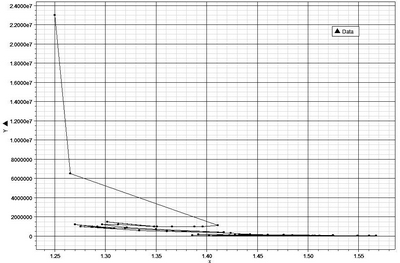Fig. 1a. The x-axis represents the range of values of gamma exhibited by the world-system over its 5000 year history, while the y-axis represents the population sizes for urban centers of maximum size per century from 3000 BCE to 2000 CE. It should be clear from this plot that the most recent values of Cmax dominate the graph obscuring the behavior of the world-system at values that are one two several orders of magnitude below those of Cmax 2000 CE and 1900 CE. However, the general shape of the plot is broadly hyperbolic in outline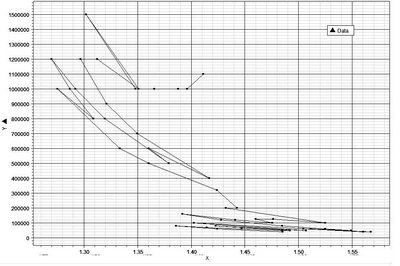Fig. 1b. The x-axis represents the range of gamma values exhibited by the world-system urban centers of maximum size over the last 4800, and the y-axis represents the actual population size of those urban centers over the same period of time. When compared with the graph in Fig. 1a the detail of the actual pattern over time is much more apparent

What is particularly revelatory about Fig. 1b is that so much of the macropattern of urbanization becomes apparent with the removal of only the final and penultimate data points, and beyond simply revealing more detail, more of the periodic behavior becomes apparent also. There are two broad periods of oscillation with one period of continuous change dividing the oscillatory periods and an on-going period of continuous change leading from the end of the second oscillatory period at 1800 CE. Also apparent within the two search pattern episodes is the fact that the second period exhibits much greater change in urban center size with a decreasing change in the absolute value of γ. However, this difference in change is most probably due to the exponential nature of the distribution function. A further note should be made here about the 12th, 13th, and 14th centuries CE. At least from a graphical perspective (see Figs 1b, 2, 3, and 4), there is no question of the uniqueness of this two hundred year period of time. The movement from the 13th Century on to the 14th Century is almost a complete reversal of the previous century's path with respect to changes in both γ and Cmax, and the following three centuries, the 15th, 16th, and 17th, show no change in Cmax but a greatly increased change in the absolute value of γ.

Elsewhere (Harper 2009), it has also been demonstrated that the relationship between γ and maximum urban center size, Cmax, is an inverse one, not surprising when again one considers that the primary equation, F = αC, shows such a relationship directly, since the exponent, gamma, is negative. However, this relationship did reveal non-obvious periodicity (see Figs 2 and 3) and also appeared to be scale free in that the same general pattern was repeated at a scale that was greater by an order of magnitude than the initial pattern. (It should be pointed out here that Korotayev  has recognized the same relationship between scale and detail of the macropattern of world-system urbanization.) Specifically, the two periods of time during which the world-system trajectory exhibited oscillations or search pattern behavior are times when the world-system population varied from approximately sixteen million to eighty million during the first period and from one hundred and sixty million to slightly greater than eight hundred million, roughly an order of magnitude change in global population. Over the same periods of time maximum urban size changed from eighty thousand to approximately one hundred thousand and from one million to slightly greater than one million respectively. Again, the difference in scale is approximately one order of magnitude, however, maximum urban size is significantly more stable and less variable than is the total population of the world-system over the same periods of time.

If the relationship between maximum urban size, Cmax, and γ is considered, rather than a of Equation 1 and γ, the same basic pattern is revealed (see Fig. 3). There are over the same periods of time episodes of oscillation followed by episodes of continuous change, and, in fact, it is quite difficult to distinguish the patterns of the two graphs. Even so, there are minor differences. For example, the sequence of values for Cmax v. γ for 1200 CE through 1400 CE has a slightly different configuration. This specific topic will be treated later in the paper. Not withstanding minor differences then, both graphs exhibit an element of scale invariance, first pointed out by Modelski (2003), and both graphs represent the same basic trends, decreasing urbanization with increasing absolute value of γ, increased urbanization with decreasing absolute value of γ, and significant historical collapses, e.g., the collapses of both the Early and Late Bronze Age associated only with episodes in which the absolute value of γ increases.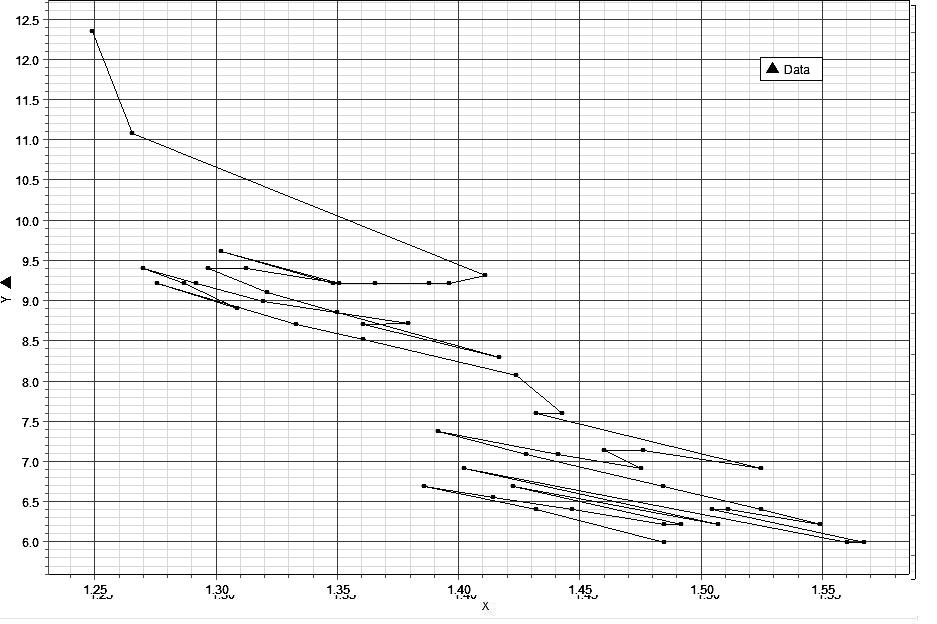Fig. 2. The x-axis represents the range of values of γ over the 5000 year history of the world-system, while the y-axis represents the range of lna, the natural logarithm of the ratio of the largest to the smallest urban area, over the same period of time. The graphical relationship represented here is both inverse and periodic, with periods of continuous increase in urban size and decrease in γ punctuated by periods of oscillation

That the relationship between γ and Cmax is an inverse one can also be shown empirically by regressing ln Cmax against γ, which gives the linear equation: ln Cmax = 33.9052 – 15.2206 γ (Equation 4). Since this equation has a negative slope, the relationship is clearly inverse. This relationship simply confirms what the fundamental assumption, F = αC, states, that as C, urban center size, increases the frequency of such centers decreases, and does so also in relation to γ, since as γ decreases, C can inversely increase with a similar effect on F. It should be noted here that while it is convenient to discuss these variables independently, the realtiy is that they interact in a coherent way to limit and define the macropattern of urbanization of the world-system over time.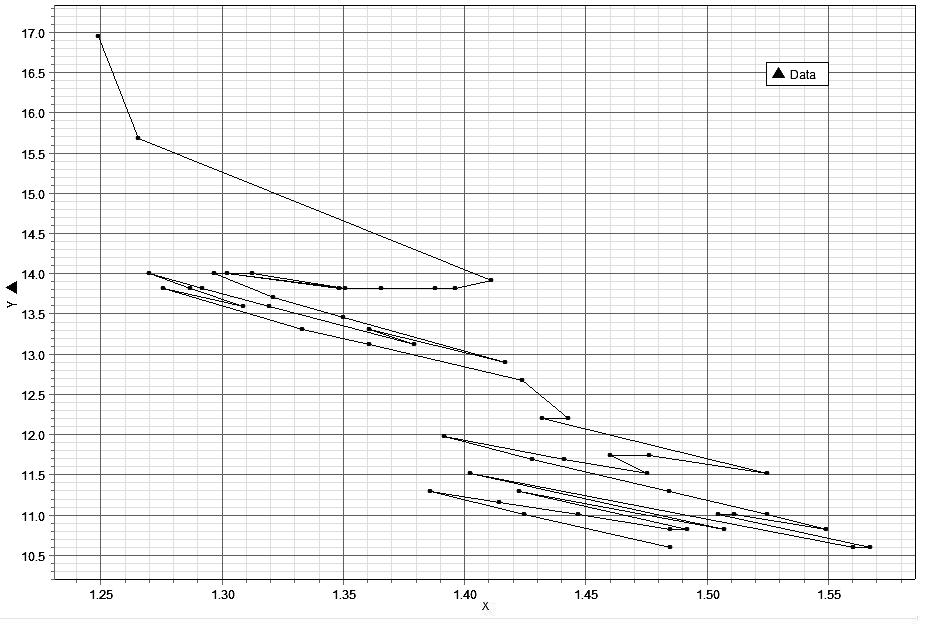Fig. 3. As in Fig. 1 the x-axis represents the range of values of γ over the 5000 year history of the world-system, while the y-axis represents the natural logarithms of population sizes of the maximum urban centers. The same broad patterns are apparent as is the scale invariant nature of the world system

The Relationship between γ and T/Cmax

One way to demonstrate interaction between the variables in Equation 2 is to represent two of these variables, T and Cmax, by defining the relationship between T and Cmax as a ratio, i.e. T/Cmax or by linearizing the ratio by taking the natural logarithm of this ratio, lnT/Cmax. It can be shown that the equilibrium value of γ is related to ln[T/Cmax] in the following way: γ = [lnT – lnlnCmax]/ln Cmax (Equation 5). (See the Matematical Appendix for the derivation of this equation.) If only the first term on the right hand side of this equation is considered, then γ ~ ln[T/lnCmax], which unquestionably represents the inverse relationship between γ and lnCmax. The relationship between γ and ln[T/Cmax] can also be represented graphically and reveals the broad characteristics exhibited by both Figs 2 and 3 (see Fig. 4). This graph clearly shows the distinction between the ancient and modern worlds and also clearly represents the disturbance of the 14th Century. What is significant about this graph is that this broad relationship holds when all three variables are represented.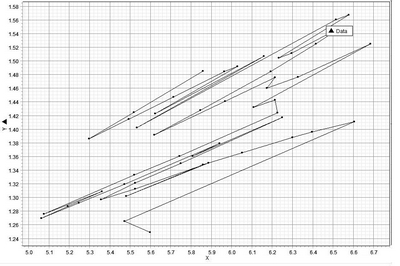Fig. 4. The x-axis represents lnT/lnCmax, and the y-axis represents γ. Note the two distinct search patterns separated by a period of 600 years and also note that the broad trend of the graph is from the upper left to the lower right, again, suggesting an inverse relationship between γ and lnCmax, and therefore Cmax

The other significant aspect of this graph is that the two episodes of oscillation are essentially scale invariant; the order of magnitude of each search pattern is approximate to the other both with respect to changes in lnCmax and in changes to γ. Specifically, ∆lnCmax is 1.28 for the Ancient World and is 1.54 for the Modern World, while ∆γ is .24 for the Ancient World and .25 for the Modern World. The similarity of scale also extends to the period of time over which each search pattern occurs, 2100 years for the Ancient World and 2200 years for the Modern World, with a period of 500–600 years separating the two phases of history and the last 200 years, 1800 CE to 2000 CE, possibly representing the beginning of the next phase of separation, i.e. the next period in which there is both a continuous decline in the absolute value of γ and a continuous increase in Cmax. If in fact the behavior of the world-system is scale invariant, this has enormous implications regarding how the world-system should be studied and what can be predicted regarding its future behavior. Scale invariance implies that the system exhibits similar characteristics and behaviors regardless of the scale of the system, i.e. that the generalized changes experienced by the Ancient World are also characteristic of the Modern World and also future states of the world-system.

The Relationship between T/Cmax and Time

The scale invariance of the system does not imply stasis, but rather that the system subject to similar stresses will behave in a similar fashion regardless of the size of the system. In light of the fact that the basic pattern of the world-system is repetitive suggests that there are one or more components of the system which are themselves cyclical or are subject to cyclical perturbations externally. Such cycles may manifest themselves as secondary trends which can be revealed by linearizing the data and then investigating the residuals (Turchin 2003). This technique will be used to investigate the existence of any secondary trends associated with T/Cmax over the 5000 year time period studied in this paper.

The data were linearized by natural logarithm transform and then a regression equation was generated using a TI-84 Plus calculator. The equation generated is: lnT/Cmax = 5.9663 – .0035t (Equation 6), where lower case ‘t’ represents time. Since the slope of this equation is barely negative, that implies that T/Cmax changes very little over time, and in fact the expected values predicted by Equation 4 exhibit a 2 % change over 5000 years. This appears to be a relatively small change in the system, and one might be tempted to suggest that the ratio, T/Cmax, is approximately constant over time. (It should be noted here that if T/Cmax increases then there is a decrease in urbanization, while a decrease in T/Cmax suggests an increase in urbanization.) There is, however, another way to look at this equation and that is by transforming it exponentially, which gives: T/Cmax = Be-.0035t (Equation 7), where B = e5.9663 or ~ 390. This equation has the form of an exponential decay curve with a small constant rate of decay, which in this case represents a small constant increase in urbanization. The exponential form of this equation predicts a change of almost 16 % in T/Cmax over time and, due to the negative exponent, suggests that there has been a relative increase in the proportion of the world-system that has been subject to urbanization over its 5000 year history.

If the analysis here is restricted to the periods 3000 BCE – 1200 BCE representing the Ancient World and 400 BCE – 1800 CE representing the (Classical and) Modern World, but excluding the most recent period of rapid change in the macropattern of world-system urbanization, the regressions for the Ancient World and the Modern World are respectively ln[T/Cmax] = .0362t + 5.6444 (Equation 8) and ln[T/Cmax] = .0351t + + 5.3425 (Equation 9). The slopes of both equations are approximately the same and are positive, the latter indicating that decreased urbanization occurs over both periods of time and does so at a similar rate. Recalling that the regression of ln[T/Cmax] over the entire period has a slope of –.0035, which implies a slight increase in urbanization. How can this disparity be accounted for since the bulk of the 5000 year period of time, 4100 years, exhibits a positive slope. The period of time from 1200 CE to 400 CE yields a regression of: ln[T/Cmax] = .0762t + 5.8982 (Equation 10), so, unquestionably, as important as this period of time is as a bridge between the Ancient and Modern Worlds, this period also exhibits a decrease in urbanization. That leaves the final two centuries of world-system history, 1800 CE – 2000 CE, however, again the slope of this two point regression is positive. The issue of course is that it is the system in toto over the entire 5000 year period that exhibits a slight negative trend. This is a clear example of what Ernst Mayer (1988) called an emergent property, i.e. a property that is an inherent characteristic of a particular level or organization within a system and not predictable per se by a reductionist approach, i.e. by analysis of the components of the level being investigated, in other words by analysis of a hier-archically lower level, which assumes that the higher level can be fully understood by this sort of component analysis.

The graph of the residuals of Equation 6 is represented in Fig. 5. It can be seen that there are four major periods of urbanization indicated by portions of the curve having a negative slope.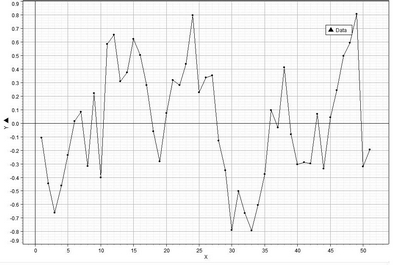Fig. 5. The x-axis represents time, and the y-axis – the residuals of ln[T/Cmax] = 5.9663 -.0035t. Periods of time exhibiting a negative slope represent periods of increased urbanization, and periods of time exhibiting a positive slope represent periods of decreased urbanization

These periods of increasing urbanization are from 1600 BCE to 1200 BCE, from 400 BCE to 100 BCE, from 700 CE to 900 CE, and from 1800 CE to 1900 CE. Interestingly, each period of increased urbanization is not always accompanied by an increase in the total population of the world-system but is always accompanied by an increase, in each case a large increase, in urbanization. See Table 1. In particular, the degree of urbanization occurring from 1800 CE to 1900 CE is vastly greater than any of the other urbanization periods not only in absolute magnitude but also with respect to change per century. Again, see Table 1. Further, when these figures are normalized to the per cent change in urbanization experienced from 1600 BCE to 1200 BCE per century the change in urbanization during the 19th Century becomes even more pronounced.

Table 1

 PERIOD % ∆T % ∆Cmax % ∆Cmax/CENT. % ∆Cmax/CENT. NORMALIZED TO 55.00 % 1600 BCE – 1200 BCE 27.93 % 220.00 % 55.00 % 1.00 400 BCE – 100 BCE –1.23 % 212.50 % 70.83 % 1.29 700 CE – 900 CE 9.18 % 125.00 % 62.5 % 1.14 1800 CE – 1900 CE 47.55 % 490.91 % 490.91 % 8.93

As is the case with regard to the pattern of urbanization there were also four major periods of deurbanization. These are from 2800 BCE to 2400 BCE, from 1200 BCE to 700 BCE, from 200 CE to 700 CE, and from 1300 CE to 1800 CE. Using the same analysis for deurbanization that was used to analyse increasing urbanization (see Table 2), it can be seen that deurbanization occurs only with decreasing Cmax, however, in each instance the population of the world-system experienced growth. The greatest period of deurbanization occurred during and after the decline and fall of the Roman Empire and the Han Empire both with regard to absolute per cent change and as normalized change, the implication being that while urbanization may be dependent on the total population of the world-system, with the exception of the time of the Roman Republic, deurbanization is not and appears to represent a reorganization of urban centers that is independent of global population size. Very definitely this pattern of deurbanization and also the pattern of urbanization are related to the formation, stability, and demise of states. Korotayev and Grinin (2010) have demonstrated quite securly the quantitative relationship between urbanization and state formation.

Table 2

 PERIOD % ∆T % ∆Cmax % ∆Cmax/CENT. % ∆Cmax/CENT. NORMALIZED TO –7.5 % 2800 BCE – 2400 BCE 29.99 % –37.50 % –9.38 % 1.25 1200 BCE – 700 BCE 81.00 % –37.50 % –7.50 % 1.00 200 CE – 700 BCE 8.95 % –200.00 % –40.00 % 5.33 1300 CE – 1800 CE 125.00 % –36.36 % –.36.36 % 4.85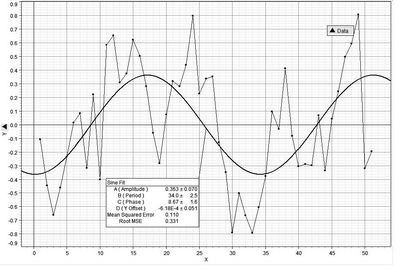Fig. 6. This graph represents the same plot as in Fig. 5 but with a best-fit sine curve. The period of this curve is approximately 3400 years, similar to those found for other regressions (see Harper 2009)

There is a broad sinusoidal trend to Fig. 5 which has been formalized in Fig. 7 with a sine curve fit yielding the equation: R = .363sin(34t + 8.67) – .000618 (Equation 11). This curve has a period of 3400 years and is similar in extent to the period of the sine fit of residuals to the equation: R = .0614sin(3410 γ + 703) + + 161 (Equation as found in Harper 2009). (Note that this curve was fitted with time in increments of 100 years, while the second equation is regressed against time as measured in years, i.e. one unit of time in Equation 11 is equivalent to 100 years in the other equation.) The root mean square error of this fit is .331. A slightly better fit is given by a sine-series fit to the same data (see Fig. 8). This curve also has a major period of approximately 3400 years, in this case 3440 years, and a minor period of 1170 years for the embedded curves within the larger sinusoidal trend.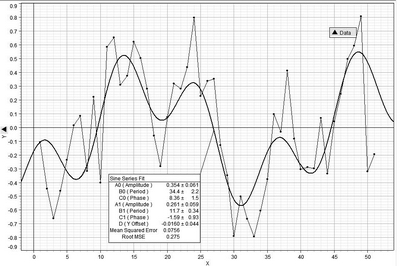Fig. 7. As in Fig. 6 this graph represents the data exhibited in Fig. 5 but with a sine-series fit. Note that the root mean square error is less with this fit than with the fit of a sine curve

This curve has a slightly better RMSE of .275. It is quite clear, however, that this second curve gives only a rough approximation of the actual trends exhibited by the data.

The Figs 5, 6, and 7 all reveal a cyclical pattern with respect to secondary trends of the regressed data on ln[T/Cmax]. This cyclicity is a potential contributor to the periodic behavior exhibited by the graphs of the world-system, although the periods of continuous change are difficult to reconcile with the periodicity of either a sine or sine-series fit. Even so there appears to be some consistency with an approximate 3400 year period. In personal communication with George Modelski he was hard pressed to account for this periodicity. However, previous remarks regarding emergent properties may have pertinence here.

A Unique Aspect of the Macropattern

A brief remark needs to be made here about what the author of popular history, Barbara Tuchman (1978), has called in the subtitle of her book, A Distant Mirror, ‘The Calamitous 14th Century’, a time in which ‘the hoofprints of more than the Four Horsemen of St. John's vision’ wrought havoc throughout this one hundred year period. While Figs 1b, 2, 3, 4 all represent in various forms the macropattern of world-system urbanization, it is quite clear that in each graph the pattern of urbanization is punctuated by the effects of the 14th Century. In each graph the course of the pattern is reversed or turns around on itself, and it is also clear that the preceeding direction of the pattern, had the 14th Century not been so calamitious, would have given this century a very different direction. In turn, the following centuries would also have taken different positions within the larger macropattern, implying that with regard to urbanization this period of time needs to be very carefully assessed. Much like the information that brain-damaged patients have yielded about the functioning of the brain, this particular time has, I believe, a similar potential.

It is interesting to observe that the general pattern established by the macropattern at this point in time is one of increase in urbanization from the 12th Century to the 13th Century but is followed by a complete reversal in path from the 13th Century to the 14th Century. This reversal takes the form of change in both variables, e.g. in Fig. 1b both lnCmax and γ show a reversal in change approximately equivalent to the previous centuries position. In other words, the reversal is an almost exact retracing of the previous century's change in position. This is also the case for the plots of the other centuries mentioned. A reasonable question to ask is: Why was the 14th Century an almost mirror image of the previous century? Also, why did the system follow this particular pathway in responding to the event of the 14th Century and not some other pathway? Further, the magnitude of Cmax remains constant in the face of an increasing absolute value of γ through the 17th Century and then shows only moderate increase to the 18th Century even as the absolute value of γ continues to grow. Why did this stasis in Cmax extend through three centuries? The answers to these questions are left to future research.

The Relative Effects of Change in γ, Cmax, and T on the Macropattern

Equation 2, Cmaxγ – Cmax – (γ – 1)T = 0, depends on three variables, γ, Cmax, and T, and it will be important to note the magnitude of effect change in each of these has on the function as a whole. This can be done by evaluating the partial derivatives of the function, here denoted as f, with respect to the three variables. These partial derivatives are: ∂f/∂γ = CmaxγlnCmax – T (Equation 12), ∂f/∂Cmax = = γCmaxγ-1 (Equation 13), and ∂f/∂T = – (γ – 1) (Equation 14). Equation 14, representing change in T, will exhibit a slight negative change that is entirely dependent on γ and will have a range between –.2499 and –.5674. It can be shown that ∂f/∂γ > ∂f/∂Cmax (see Mathematical Appendix), and since ∂f/∂Cmax > 1, then ∂f/∂γ > > ∂f/∂Cmax > ∂f/∂T. What this implies is that a change in γ will have the greatest effect on the system, followed by a change in Cmax followed by changes in T, and this is what the data seem to imply, especially with respect to de-urbanization.

Summary

The macropattern of urbanizaton exhibits considerable regularity, regularity that is a consequence of interaction between γ, Cmax, and T. There are both periods of continuous increase in urbanization and periods in which urbanization and de-urbanization fluctuate over time. The following characteristics have been discussed:

1. An unscaled graphical representation of the data reveals the inverse relationship between γ and Cmax, however, much of the detail of the pattern is not apparent.

2. By removing the last two data points from this plot, the non-linear detail becomes much more apparent. This has two implications, that the urbanization of the last two centuries has had an over-riding effect on the world-system, and that the previously obscured data suggest at least two periods of oscillation in which the values of γ and Cmax fluctuate.

3. By transforming the data on Cmax with natural logarithms the finer scaled behavior of the world-system pattern of urbanization, occurring at lower values of Cmax and T and higher ones for γ, becomes apparent.

4. A linear regression of γ and lnCmax yields a negative slope supporting the inverse relationship between these two variables.

5. If the ratio of T/Cmax is considered with respect to γ and the data for T/Cmax is linearized by natural logarithm transform, a regression of the entire, linearized data set yields a negative slope which implies continued increase of Cmax with respect to T over time, i.e. the ratio decreases. This also implies an inverse relationship between γ and T/Cmax.

6. The graph of γ and ln[T/Cmax] clearly reveals two broad search patterns or patterns of oscillation separated by an extended period in which the absolute value of γ decreases, implying increased maximum urban center size.

7. The graph of γ and ln[T/Cmax] also reveals the essential scale invariance of the two search pattern periods. This has significant implication not only for analyzing the past behavior of the world-system with respect to urbanization but also with respect to predictions about the future behavior of the world-system.

8. If regressions of various portions of the urbanization macropattern are considered, e.g. from 3000 BCE to 1200 BCE, 1200 BCE to 400 BCE, and 400 BCE to 1800 BCE, the regressions all yield positive slopes. It is suggested that the slight negative slope of the entire system is an emergent property of the system.

9. The residuals of the regression of the world-system over the entire 5000 year period reveal a roughly cyclical pattern, one that is only reasonably well modeled by both a sine fit and a sine series fit of the data.

10. The graph of the residuals of the regression, lnT/Cmax = = 5.9663 – .0035t, reveal four periods of increased urbanization and four periods of decreased urbanization. The period of greatest increase in urbanization is the one we are currently experiencing, and the period of greatest decrease in urbanization is coincident with the demise of both the Roman and Han Empires and the succeeding centuries labeled as Dark Ages.

11. A unique aspect of the macropattern is the period of time encompassing the 12th through the 14th centuries. It is quite clear that the previous pattern of urbanization is disrupted and also quite clear that urbanization exhibits stasis for the next three hundred years.

12. An analysis of the partial derivatives of Equation 2 reveals that change in γ will have the greatest effect on world-system urbanization, followed by change in Cmax, with the least effect being caused by a change in T.

Acknowledgements

I wish to thank both Andrey Korotayev and Leonid Grinin for their encouragement to write this paper. However, I take full responsibility for any errors within.

Note

* The paper is dedicated to the scholars of the Russian School of Cliodynamics who opened the door for the rest of us.

Mathematical Appendix

Derivation of Equation 2.

Equation 2, Cmaxγ – Cmax – (γ – 1)T = 0, can be easily derived from Equation 1, aγC0γ-1 – a – (γ – 1)T/C0 = 0, in the following way: Since Cmax = a C0 as defined in Harper (in print) then by setting aγC0γ-1 – a = (γ – 1)T/C0 and multiplying both sides by C0 gives: aγC0γ – aC0 = (γ – 1)T, which then converts to: Cmaxγ – Cmax = = (γ – 1)T or Cmaxγ – Cmax – (γ – 1)T = 0.

The Derivation of γ = f(T/ Cmax).

Since f(γ, Cmax, T) = Cmaxγ – Cmax – (γ – 1)T = 0, then ∂f/∂γ = = Cmaxγln Cmax – T = 0. Then Cmaxγln Cmax = T, and transforming by natural logarithms gives: γ lnCmax + lnlnCmax = lnT. So, γ = [lnT – – lnlnCmax]/ln Cmax, which implies that γ ~ lnT/ lnCmax, since [lnT – – lnlnCmax]/lnCmax = [lnT/ lnCmax] – [lnlnCmax/ lnCmax].

Proof of ∂f/∂γ > ∂f/∂Cmax > ∂f/∂T.

If ∂f/∂γ > ∂f/∂Cmax, then CmaxγlnCmax – T > γCmaxγ-1, and CmaxγlnCmax – γCmaxγ-1 > T – 1. Since T is at least seven orders of magnitude greater than 1, then CmaxγlnCmax – – γCmaxγ-1 > T. From Equation 2, T = [Cmaxγ – Cmax]/[γ – 1], then CmaxγlnCmax – γCmaxγ-1 > [Cmaxγ – Cmax]/[γ – 1], then CmaxγlnCmax – γCmaxγ-1 – Cmaxγ/(γ – 1) > – Cmax/(γ – 1). It follows that Cmaxγ [lnCmax – γCmaxγ-2 – 1/(γ – 1)] > – Cmax/(γ – 1), and then Cmaxγ > – – Cmax/{(γ – 1) [lnCmax – γCmaxγ-2 – 1/(γ – 1)]}. Since for Cmax > 1 E 4, as determined empirically, since 1.2 < γ < 1.6, then Cmaxγ-2 < 1, γCmaxγ-2 < γ, and 1.6 < [1/(γ – 1)] < 5. Consequently, since lnCmax > 9, which implies that {(γ – 1) [lnCmax – γCmaxγ-2 – 1/(γ – 1)]} > 0, then ∂f/∂γ > ∂f/∂Cmax. Also, since ∂f/∂Cmax = γCmaxγ-1, and since Cmax > 1 E 4, 1.2 < γ < 1.6, which implies .2 < γ – 1 < .6, then Cmaxγ-1 > 250, as is γCmaxγ-1, which implies that ∂f/∂Cmax > 0, so ∂f/∂γ > ∂f/∂Cmax > ∂f/∂T.

REFERENCES

Chandler, T.

1987. Four Thousand Years of Urban Growth. New York: Edwin Mellen Press.

Harper, A.

2009. The Trajectory of the World-System over the Last 5000 Years. In print.

Korotayev, A.

2010. The World-System Urbanization Macrodynamics: A Quantitative Analysis. In Malkov, S., Grinin, L., and Korotayev, A. (eds.), History and Mathematics: Evolutionary Historical Macrodynamics (pp. 23–42). Moscow: Librocom.

Korotayev, A., and Grinin, L.

2010. Political Development of the World-System and Urbanization: A Comparative Quantitative Analysis. In Malkov, S., Grinin, L., and Korotayev, A. (eds.), History and Mathematics: Evolutionary Historical Macrodynamics (pp. 119–188). Moscow: Librocom.

Mayer, E.

1988. Toward a New Philosophy of Biology: Observations of an Evolutionist. Cambridge, Mass.: Belknap Press of Harvard University Press.

Modelski, G.

2003. World Cities: –3000 to 2000. New York: FAROS 2000.

U.S. Census Bureau.

2009. Historical Estimates of World Population. Available at: http://www.census. gov/ipc/www/worldhis.html

Tuchman, B.

1978. A Distant Mirror: The Calamitous 14th Century. New York: Ballantine Books.

Turchin, P.

2003. Historical Dynamics: Why States Rise and Fall. Princeton: Princeton University Press.

31.10.2023

24.10.2023

10.08.2023